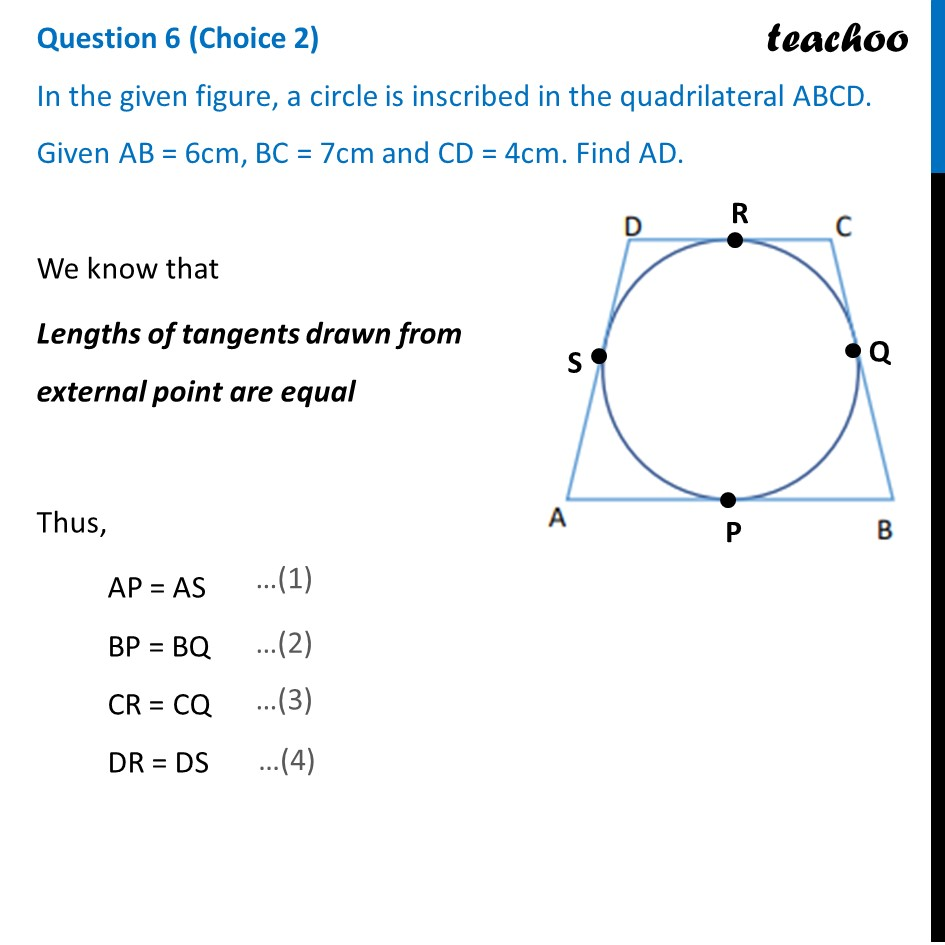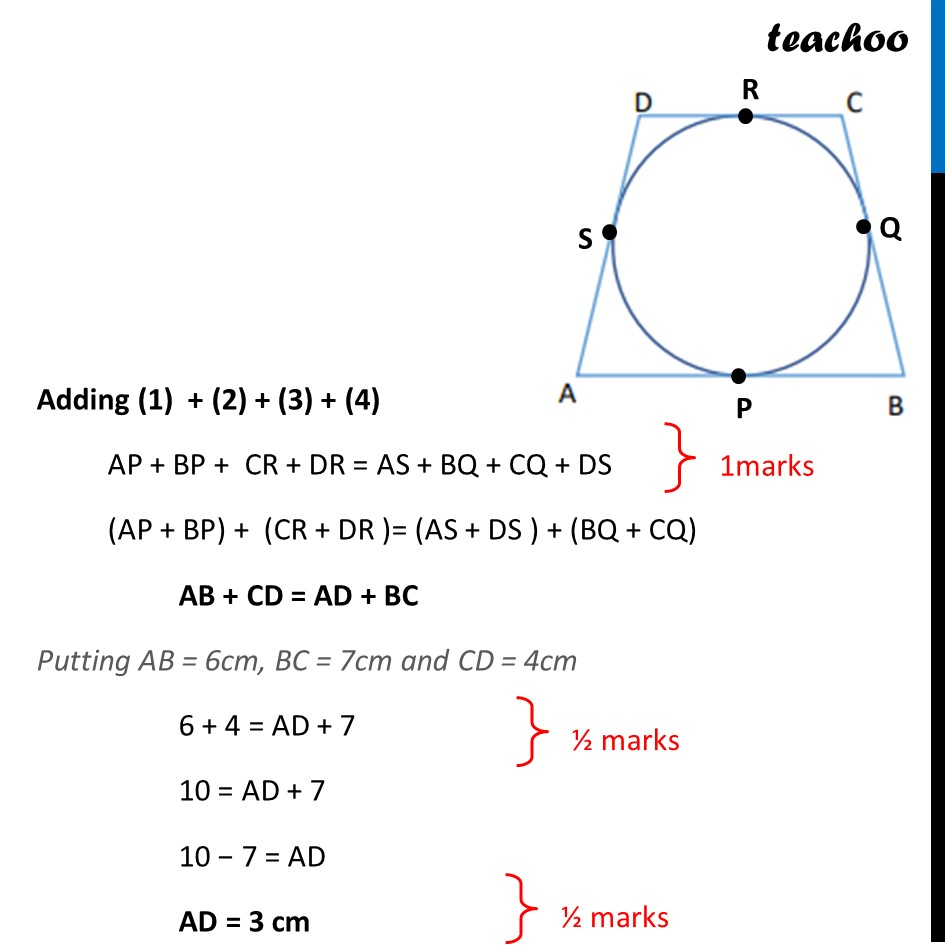CBSE Class 10 Sample Paper for 2022 Boards - Maths Basic [Term 2]

Class 10
Solutions of Sample Papers for Class 10 Boards

## In the given figure, a circle is inscribed in the quadrilateral ABCD. Given AB = 6cm, BC = 7cm and CD = 4cm. Find AD.

This question is similar to Ex 10.2, 8 Chapter 10 Class 10 - CirclesLearn in your speed, with individual attention - Teachoo Maths 1-on-1 Class

### Transcript

In the given figure, a circle is inscribed in the quadrilateral ABCD. Given AB = 6cm, BC = 7cm and CD = 4cm. Find AD. We know that Lengths of tangents drawn from external point are equal Thus, AP = AS BP = BQ CR = CQ DR = DS Adding (1) + (2) + (3) + (4) AP + BP + CR + DR = AS + BQ + CQ + DS (AP + BP) + (CR + DR )= (AS + DS ) + (BQ + CQ) AB + CD = AD + BC Putting AB = 6cm, BC = 7cm and CD = 4cm 6 + 4 = AD + 7 10 = AD + 7 10 − 7 = AD AD = 3 cm